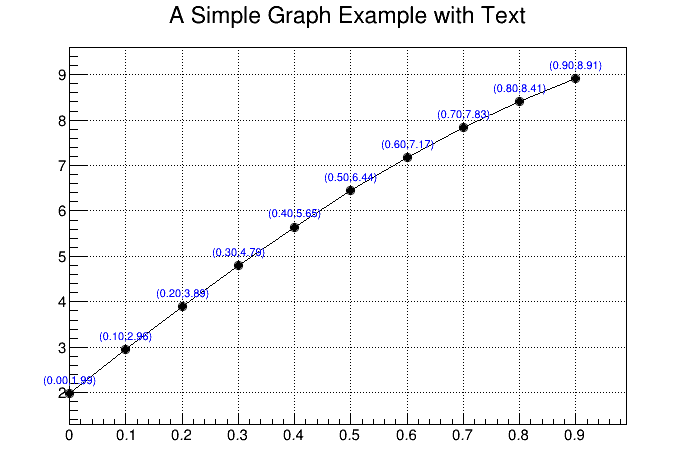# graphtext¶

Draw a graph with text attached to each point. The text is drawn in a TExec function attached to the TGraph, therefore if the a graph's point is moved interactively, the text will be automatically updated.

Author: Olivier Couet
This notebook tutorial was automatically generated with ROOTBOOK-izer from the macro found in the ROOT repository on Sunday, October 02, 2022 at 09:31 AM.

Definition of a helper function:

In :
%%cpp -d
void drawtext()
{
Int_t i,n;
Double_t x,y;
TLatex l;

l.SetTextSize(0.025);
l.SetTextFont(42);
l.SetTextAlign(21);
l.SetTextColor(kBlue);

auto g = (TGraph*)gPad->GetListOfPrimitives()->FindObject("Graph");
n = g->GetN();

for (i=0; i<n; i++) {
g->GetPoint(i,x,y);
l.PaintText(x,y+0.2,Form("(%4.2f,%4.2f)",x,y));
}
}

In :
TCanvas *c = new TCanvas("c","A Simple Graph Example with Text",700,500);
c->SetGrid();

const Int_t n = 10;
auto gr = new TGraph(n);
gr->SetTitle("A Simple Graph Example with Text");
gr->SetMarkerStyle(20);
auto ex = new TExec("ex","drawtext();");

Double_t x, y;
for (Int_t i=0;i<n;i++) {
x = i*0.1;
y = 10*sin(x+0.2);
gr->SetPoint(i,x,y);

}
gr->Draw("ALP");


Draw all canvases

In :
gROOT->GetListOfCanvases()->Draw()